# Introduction to the Grating Equation and Grating Physics

Diffraction gratings are used for dispersing light, i.e., for spatially separating light of different wavelengths. They are used as an alternative to prisms in most fields of spectral analysis. Their production dates back to the late eighteenth century. Extensive adoption was limited by the challenges in ruling gratings of adequate quality until the development of ruling engines by Henry Rowland in the late 19th century.

The subsequent manufacture of high quality gratings revolutionized analytical spectroscopy. At present, most diffraction gratings are reproduced from masters, which can be ruled with interferometric control, produced holographically with possible improvement by means of ion etching or advanced techniques semiconductor lithography.

## The Grating Equation

A typical diffraction grating comprises a substrate of an optical material with numerous parallel grooves ruled or reproduced on its surface. The substrate is coated with a reflecting material like aluminum. The quality and spacing of the grooves are significant to the performance of the grating. Nevertheless, the basic grating equation may be obtained by assuming a section through the grating surface at right angle to the ruling direction as a sawtooth pattern (Figure 1).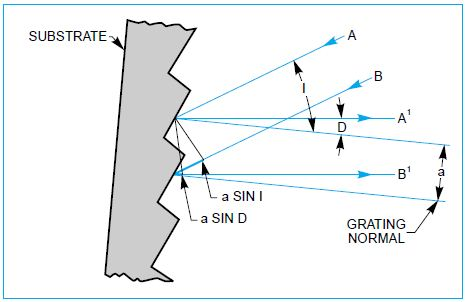Figure 1. The sawtooth pattern of a grating section.

Figure 1 shows the incident of light rays A and B with a wavelength of λ on adjacent grooves at angle I to the grating normal. If the light is considered at angle D to the grating normal, then it originates from the A and B rays when they hit the grating. Then the path difference between the A1 and B1 rays is a sin I + a sin D. Adding the A1 and B1 rays gives rise to constructive interference when the path difference is the same of any integer multiple of the wavelength λ. This yields the basic grating equation as follows:

a(sin I + sin D) = mλ

Where, m = an integer, and is the order of diffraction.

## The Grating Equation in Practice

The incident of a parallel beam of monochromatic light on a grating causes diffraction of the light from the grating in directions relative to m = -2, -1, 0, 1, 2, 3, etc (Figure 2). Conversely, the incident of a parallel beam of polychromatic light on a grating causes dispersion of the light, meaning that each wavelength follows the grating equation, as shown in Figure 3.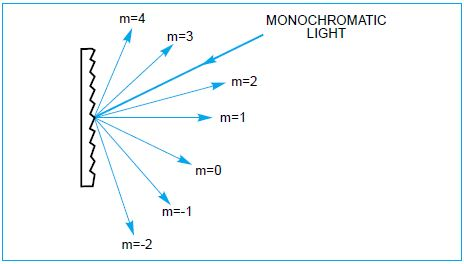Figure 2. The “Grating Equation” satisfied for a parallel beam of monochromatic light.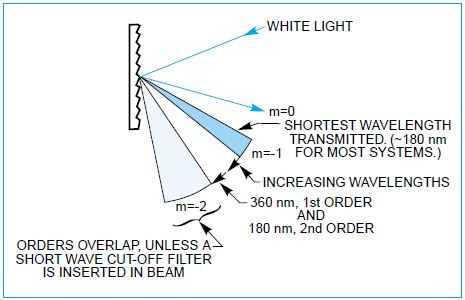Figure 3. Polychromatic light diffracted from a grating. Positive orders have been omitted for clarity.

For use with monochromators, the grating equation can be expressed as:

Mλ = 2 × a × cos φ × sin θ

Where, φ = Half the included angle between the incident ray and the diffracted ray at the grating, and θ = Grating angle relative to the zero order position.

The relation between these terms and the incident angle I and diffracted angle D can be expressed as follows:

I = θ + φ and D = θ – φ

It is to be noted that the sign of ‘m’ is provided by either form of the grating equation and can be positive or negative. The rotational position of the grating determines the angles I and D in a monochromator. The incident light, grating rotation, and diffracted light can be at positive or negative angles based on which side of the grating normal they are. The half angle is always considered as positive (Figure 4).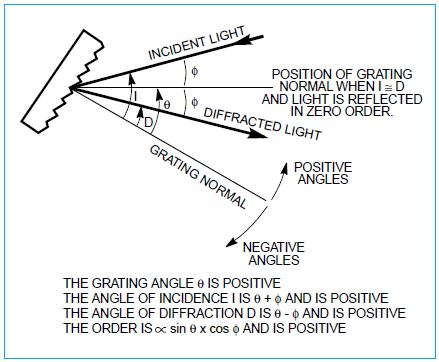Figure 4. The sign convention for the angle of incidence, angle of diffraction and grating angle.

The grating equation is also fulfilled for wavelengths in higher orders, when | m | is >1. Usually only the first order, either negative or positive, is preferred. It may be necessary to block other higher order wavelengths. The input spectrum and detector sensitivity decide the requirement of employing order sorting or blocking filters.

## Blaze Wavelength

The incident of monochromatic light on a grating causes diffraction of a fraction of the light into each other. The fraction diffracted into any order can be called the efficiency of the grating in that order. All Oriel Gratings are engineered for efficient diffraction into the first order. The optimization of efficiency by correct groove shaping is called blazing and the wavelength at which the grating exhibits optimum efficiency is called ‘Blaze Wavelength.’ A typical efficiency vs. wavelength curve for a ruled and a blazed holographic grating is illustrated in Figure 5.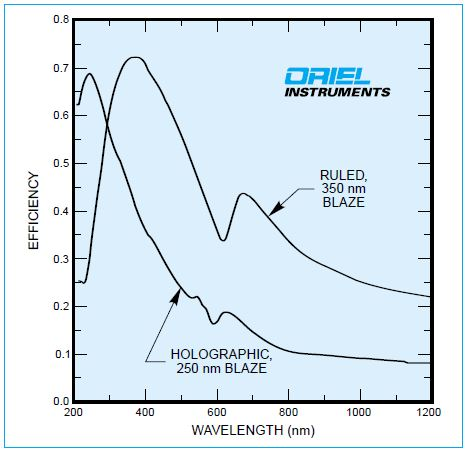Figure 5. The efficiency of a ruled and a blazed holographic grating, both have 1200 l/mm.

## Holographic Gratings

Holographic gratings are fabricated using a sinusoidal interferometric pattern and at times using an etching process. Sinusoidally grooved gratings yield very little scattered light but have low, flat efficiency curves. Blazed holographic gratings employ etching in the interferometric process, or an ion gun to create a blaze angle in a secondary process. Oriel Instruments provides both ruled and holographic gratings to yield unprecedented efficiency, blaze, and low light scatter for various wavelength ranges.

## Dispersion

If the angle I in the grating equation is fixed and differentiated with respect to wavelength, then:

a × cos D × δD = m × δλ

thus: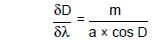Where, δD/δλ is the angular dispersion or change of diffraction angle relative to a slight change in wavelength. It is higher for smaller groove spacing, a (greater number of lines per millimeter); larger orders, m; and larger diffraction angles, D.

The linear dispersion, δL/δλ, at the exit slit of a monochromator will change with output focal length, f, and angle D and is the product of the focal length and angular dispersion. For Oriel 77250 Monochromator and 77700 Monochromator and Spectrograph each with 1200 l/mm gratings, the linear dispersion is: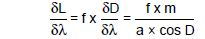## Bandpass and Resolution

The width of a spectrum passed by a spectrograph or monochromator when subjected to illumination by a light source of continuous spectrum is called the bandpass. Decreasing the width of the slits until reaching a limiting bandpass may decrease the bandpass in monochromators. The limiting bandpass is known as the resolution of the device. In spectral analysis, the resolution of the instrument is a measure of its ability to isolate two closely positioned spectral lines.

## Grating Illumination and Resolution

The resolving power ‘R’ of a grating is expressed in terms of wavelengths λ and λ + δλ, where λ + δλ is the closest wavelength to λ which can be resolved. The resolving power ‘R’ can be theoretically expressed as: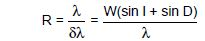Where, δλ is the resolution and ‘W’ is the illuminated width of the grating. Generally, the smallest slit available and optical aberrations, instead of the grating, determine the achievable resolution.

## Double Dispersion and Stray Light

If two monochromators are employed in tandem, the reciprocal linear dispersion of the combination is 50% of that of a single monochromator. The exit beam from a standard monochromator typically consists of unwanted light of other wavelengths. The use of filters can block higher order diffracted wavelengths, but other undesired light, known as stray light, has numerous origins and must be reduced.

Generally, part of this undesired light, known as re-entrant spectra, is caused by diffracted light being directed onto the entrance, collimating or focusing mirror, and returns to the grating. Additionally, the reflection of a light off a focal plane detector can also result in re-entrant spectra. The use of the Ebert-Fastie out of plane design eliminates re-entrant spectra in the 77250 Monochromator and the 77700 Monochromator. In the 77400 Spectrograph, re-entrant spectra are absorbed by tilting the detector and on baffles. The use of a double monochromator greatly improves the signal/stray light ratio.This information has been sourced, reviewed and adapted from materials provided by Oriel Instruments.

## Citations

• APA

Oriel Instruments. (2019, July 25). Introduction to the Grating Equation and Grating Physics. AZoM. Retrieved on October 23, 2020 from https://www.azom.com/article.aspx?ArticleID=10819.

• MLA

Oriel Instruments. "Introduction to the Grating Equation and Grating Physics". AZoM. 23 October 2020. <https://www.azom.com/article.aspx?ArticleID=10819>.

• Chicago

Oriel Instruments. "Introduction to the Grating Equation and Grating Physics". AZoM. https://www.azom.com/article.aspx?ArticleID=10819. (accessed October 23, 2020).

• Harvard

Oriel Instruments. 2019. Introduction to the Grating Equation and Grating Physics. AZoM, viewed 23 October 2020, https://www.azom.com/article.aspx?ArticleID=10819.